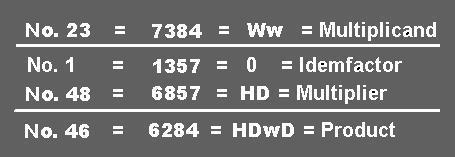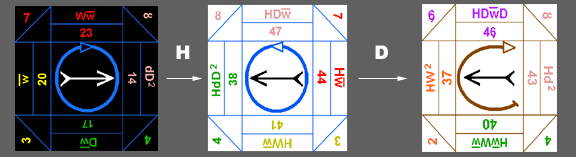Here, the Multiplier is 6857 =HD, which signifies a horizontal reflection (H) of the Multiplicand's Exact Face and then a major Deosil turn (D) from that Exact Face. The Algorithm is set up as before to calculate the result of the Product.The visual demonstration of the above Tropic Algorithm is shown first as a horizontal reflection or "mirroring" (H) from the Convex to Concave tablock, followed by a major Deosil turn (D). See the illustration below.As always, the Product of each Algorithm should be demonstrated with the physical tablocks to verify correctness between thought & action. Alternately, the virtual tablocks may be used online but it is recommended that a proper physical set be created for use.

NEXT